# FIR Interpolator

Finite impulse response (FIR) interpolation filter

Since R2022a

•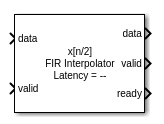Libraries:
DSP HDL Toolbox / Filtering

## Description

The FIR Interpolator block implements a single-rate polyphase FIR interpolation filter that is optimized for HDL code generation. The block provides a hardware-friendly interface with input and output control signals. To provide a cycle-accurate simulation of the generated HDL code, the block models architectural latency including pipeline registers and resource sharing.

The block accepts scalar or vector input and outputs a scalar or vector depending on the interpolation factor and the number of cycles between input samples. The block implements a polyphase decomposition with InterpolationFactor subfilters. The filter can implement a serial architecture if there is regular spacing between input samples.

The block provides two filter structures. The direct form systolic architecture provides an implementation that makes efficient use of Intel® and Xilinx® DSP blocks. This architecture can be fully-parallel or serial. To use a serial architecture, the input samples must be spaced out with a regular number of invalid cycles between the valid samples. The direct form transposed architecture is a fully parallel implementation that is suitable for FPGA and ASIC applications. For a filter implementation that matches multipliers, pipeline registers, and pre-adders to the DSP configuration of your FPGA vendor, specify your target device when you generate HDL code.

All filter structures optimize hardware resources by sharing multipliers for symmetric or antisymmetric filters and by removing the multipliers for zero-valued coefficients such as in half-band filters and Hilbert transforms.

## Ports

### Input

expand all

Input data, specified as a real or complex scalar or vector. The vector size must be less than or equal to 64. When the input data type is an integer type or a fixed-point type, the block uses fixed-point arithmetic for internal calculations.

The software supports `double` and `single` data types for simulation, but not for HDL code generation.

Data Types: `fixed point` | `single` | `double` | `int8` | `int16` | `int32` | `uint8` | `uint16` | `uint32`
Complex Number Support: Yes

Control signal that indicates if the input data is valid. When valid is `1` (`true`), the block captures the values from the input data port. When valid is `0` (`false`), the block ignores the values from the input data port.

Data Types: `Boolean`

Control signal that clears internal states. When reset is `1` (`true`), the block stops the current calculation and clears internal states. When the reset is `0` (`false`) and the input valid is `1` (`true`), the block captures data for processing.

For more reset considerations, see the Reset Signal section on the Hardware Control Signals page.

#### Dependencies

To enable this port, on the Control Ports tab, select Enable reset input port.

Data Types: `Boolean`

### Output

expand all

Interpolated output data, returned as a real or complex scalar or vector. The vector size is InputSize * InterpolationFactor. When NumCycles is greater than InterpolationFactor, scalar output samples are spaced with `floor(NumCycles/InterpolationFactor)` invalid cycles, and the output valid signal indicates which samples are valid after interpolation.

When the input data type is a floating-point type, the output data inherits the data type of the input data. When the input data type is an integer type or a fixed-point type, the Output parameter on the Data Types tab controls the output data type.

Data Types: `fixed point` | `single` | `double` | `int8` | `int16` | `int32` | `uint8` | `uint16` | `uint32`
Complex Number Support: Yes

Control signal that indicates if the data from the output data port is valid. When valid is `1` (`true`), the block returns valid data from the output data port. When valid is `0` (`false`), the values from the output data port are not valid.

Data Types: `Boolean`

Control signal that indicates that the block is ready for new input data sample on the next cycle. When ready is `1` (`true`), you can specify the data and valid inputs for the next time step. When ready is `0` (`false`), the block ignores any input data in the next time step.

Data Types: `Boolean`

## Parameters

expand all

### Main

FIR filter coefficients, specified as a real- or complex-valued vector. You can specify the vector as a workspace variable or as a call to a filter design function. When the input data type is a floating-point type, the block casts the coefficients to the same data type as the input. When the input data type is an integer type or a fixed-point type, you can set the data type for the coefficients on the Data Types tab.

Example: `firpm(30,[0 0.1 0.2 0.5]*2,[1 1 0 0])` defines coefficients by using a linear-phase filter design function.

Complex Number Support: Yes

Specify the HDL filter architecture as one of these structures:

• `Direct form systolic` — This architecture provides a parallel or partly serial filter implementation that makes efficient use of Intel and Xilinx DSP HDL blocks. For a partly serial implementation, specify a value greater than 1 for the Minimum number of cycles between valid input samples parameter. You cannot use frame-based input with the partly serial architecture.

• `Direct form transposed` — This architecture is a fully parallel implementation that is suitable for FPGA and ASIC applications.

The block implements a polyphase decomposition filter by using Discrete FIR Filter blocks. Each filter phase shares resources internally where coefficients and serial options allow. For architecture details, see FIR Filter Architectures for FPGAs and ASICs. When you use a partly-serial systolic architecture and Minimum number of cycles between valid input samples is larger than the filter length divided by the interpolation factor, the block interleaves each phase of coefficients over a single FIR filter to share resources between phases.

Specify an integer interpolation factor greater than two. The output vector size is InputSize * InterpolationFactor. The output vector size must be less than 64 samples.

Serialization requirement for input timing, specified as a positive integer. This parameter represents N, the minimum number of cycles between valid input samples. When you set Minimum number of cycles between valid input samples greater than the filter length, L, and the input and coefficients are both real, the filter uses a single multiplier.

Because the block applies coefficient optimizations before serialization, the sharing factor of the final filter can be lower than the number of cycles that you specified.

#### Dependencies

To enable this parameter, set Filter structure to `Direct form systolic`.

You cannot use frame-based input with Minimum number of cycles between valid input samples greater than 1.

### Data Types

Rounding mode for type-casting the output to the data type specified by the Output parameter. When the input data type is floating point, the block ignores this parameter. For more details, see Rounding Modes.

Overflow handling for type-casting the output to the data type specified by the Output parameter. When the input data type is floating point, the block ignores this parameter. For more details, see Overflow Handling.

The block casts the filter coefficients to this data type. The quantization rounds to the nearest representable value and saturates on overflow. When the input data type is floating point, the block ignores this parameter.

The recommended data type for this parameter is ```Inherit: Same word length as input```.

The block returns a warning or error if either of these conditions occur.

• The coefficients data type does not have enough fractional length to represent the coefficients accurately.

• The coefficients data type is unsigned, and the coefficients include negative values.

The block casts the output of the filter to this data type. The quantization uses the settings of the Rounding mode and Overflow mode parameters. When the input data type is floating point, the block ignores this parameter.

The block increases the word length for full precision inside each filter tap and casts the final output to the specified type. The maximum final internal data type (WF) depends on the input data type (WI), the coefficient data type (WC), and the number of coefficients (L) and is given by

```WF = WI + WC + ceil(log2(L))```.

Because the coefficient values limit the potential growth, usually the actual full-precision internal word length is smaller than WF.

### Control Ports

Select this check box to enable the reset input port. The reset signal implements a local synchronous reset of the data path registers.

For more reset considerations, see the Reset Signal section on the Hardware Control Signals page.

Select this check box to connect the generated HDL global reset signal to the data path registers. This parameter does not change the appearance of the block or modify simulation behavior in Simulink®. When you clear this check box, the generated HDL global reset clears only the control path registers. The generated HDL global reset can be synchronous or asynchronous depending on the HDL Code Generation > Global Settings > Reset type parameter in the model Configuration Parameters.

For more reset considerations, see the Reset Signal section on the Hardware Control Signals page.

## Algorithms

expand all

The block implements a polyphase filter bank where the filter coefficients are decomposed into InterpolationFactor subfilters. If the filter length is not divisible by the Interpolation factor parameter value, then the block zero-pads the coefficients. When your input is regularly spaced, with two or more cycles between valid samples, as indicated by the Minimum number of cycles between valid input samples parameter, the filter can share multiplier resources in time.

This flow chart shows which filter architectures result from your parameter settings. It also shows the number of multipliers used by the filter implementation. The filter architecture depends on the input frame size, V, the interpolation factor, R, the number of cycles between valid input samples, N, and the number of filter coefficients, L. The architectures are in order from lowest resource use on the left, to higher resources on the right. The higher resource architectures are trading off resource use for higher throughput. Each architecture is described below the flow chart.

The number of multipliers shown in the flow chart is for filters with real input and real coefficients. For complex input, the filter uses three times as many multipliers.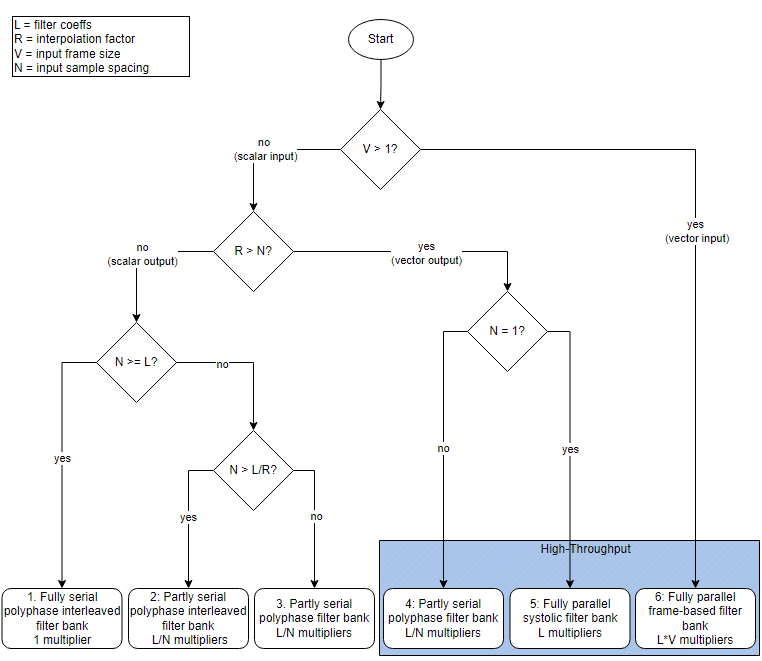• Architectures 1 and 2 — Serial polyphase interleaved filter bank.

When NumCycles is greater than FilterLength/InterpolationFactor, the block interleaves each phase of coefficients over a single FIR filter to share resources between phases. The single FIR filter has a serial architecture and uses the NumCycles value to share multipliers over time. A partly serial filter uses FilterLength/NumCycles multipliers. When NumCycles is greater than the filter length, the filter becomes fully serial and uses one multiplier. The diagram shows a filter with an interpolation factor of 4 and at least FilterLength/4 cycles between input samples.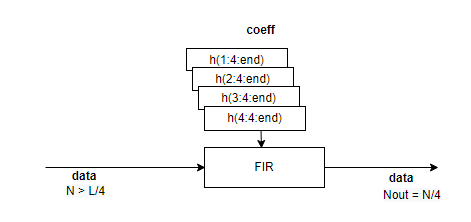• Architectures 3 and 4 — Partly serial polyphase filter bank.

Each subfilter of the polyphase decomposition uses the NumCycles value to implement a serial filter. When NumCycles is FilterLength/InterpolationFactor, each phase uses a single multiplier, for a total of InterpolationFactor multipliers. The output is a vector if NumCycles < InterpolationFactor and a scalar if NumCyclesInterpolationFactor.

The diagram shows a polyphase filter bank with scalar input and InterpolationFactor is set to 4. NumCycles is 2. Each subfilter is partly serial and has FilterLength/(4*2) multipliers, for a total of FilterLength/2 multipliers. The output is a vector because the interpolation factor is larger than the number of cycles between input samples.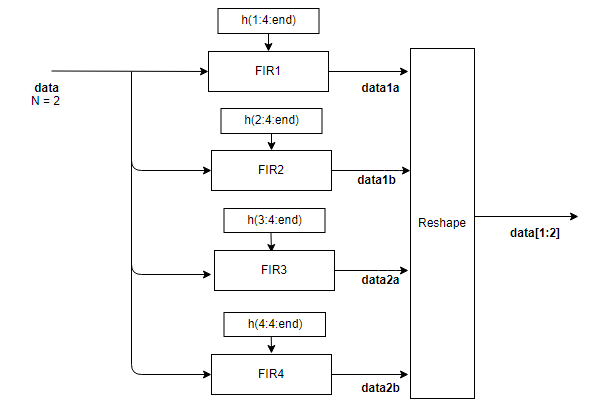• Architectures 5 and 6 — Fully parallel polyphase filter bank.

The diagram shows the polyphase filter bank with scalar input and InterpolationFactor is set to 4. The NumCycles value is 1, so the output must be a vector and each subfilter contributes one sample to the output vector. Each subfilter is a fully parallel FIR filter, with L/4 multipliers, for a total of L multipliers.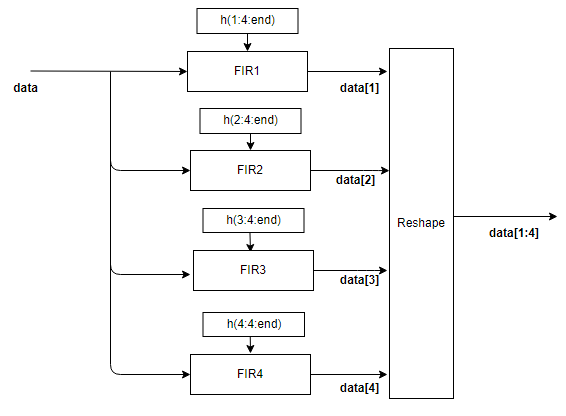When the input is a vector, each subfilter is a frame-based filter that contains one parallel filter for each input sample. This diagram shows the polyphase filter bank for an input vector of two values and InterpolationFactor is set to 4. Each of the four subfilters generates two samples of the output vector and contains L/4*2 multipliers, for a total of L*2 multipliers.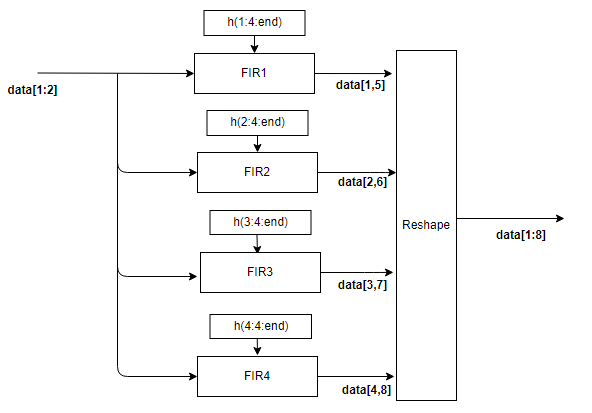Each subfilter is implemented with a Discrete FIR Filter block. For architecture details, see FIR Filter Architectures for FPGAs and ASICs.

## Version History

Introduced in R2022a

expand all